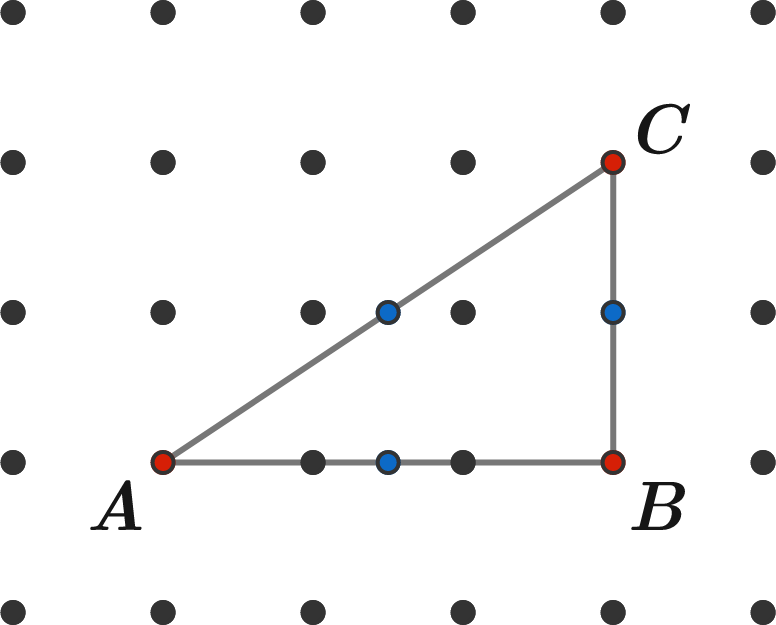# Avoid lattice midpoints

Geometry Level 2

You select a set of $N$ specific points in the 2D plane with integer coordinates such that, for every pair of the $N$ points, the midpoint of the segment connecting them does not have integer coordinates.

What is the greatest possible value of $N?$

Below is an invalid selection of 3 points (in red), since the midpoint of $BC$ has integer coordinates.×